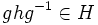# Normality satisfies transfer condition

This article gives the statement, and possibly proof, of a subgroup property satisfying a subgroup metaproperty
View all subgroup metaproperty satisfactions | View all subgroup metaproperty dissatisfactions |Get help on looking up metaproperty (dis)satisfactions for subgroup properties
|

This article gives the statement, and possibly proof, of a basic fact in group theory.
View a complete list of basic facts in group theory
VIEW FACTS USING THIS: directly | directly or indirectly, upto two steps | directly or indirectly, upto three steps|

## Statement

### Verbal statement

If a subgroup is normal in the group, its intersection with any other subgroup is normal in that subgroup.

### Symbolic statement

Let$H \le G$ be a normal subgroup and let$K$ be any subgroup of$G$. Then,$H \cap K \triangleleft K$.

### Property-theoretic statement

The subgroup property of being normal satisfies the transfer condition.

## Definitions used

### Normal subgroup

A subgroup$H$ of a group$G$ is said to be normal if for any$g \in G$ and$h \in H$,$ghg^{-1} \in H$.

### Transfer condition

A subgroup property$p$ is said to satisfy transfer condition if whenever$H, K$ are subgroups of$G$ and$H$ has property$p$ in$G$,$H \cap K$ has property$p$ in$K$.

## Related facts

### Further facts

• Second isomorphism theorem: This result equates the quotient of the non-normal subgroup, by the intersection, with the quotient of the product of subgroups, by the normal subgroup. Specifically, it states that if$H$ is normal in$G$ and$K$ is any subgroup of$G$, we have$H/(H \cap K) \cong HK/K$.

### Related metaproperties satisfied by normality

• Normality satisfies intermediate subgroup condition: The intermediate subgroup condition is weaker. It says that if$H \le K \le G$ are subgroups are$H$ is normal in$G$, then$H$ is normal in$K$.
• Normality satisfies inverse image condition: The inverse image condition is stronger. It says that the inverse image of a normal subgroup under a homomorphism is normal.

Other metaproperties satisfied by normality, that are somewhat related:

## Proof

### Hands-on proof

Given: A group$G$, a normal subgroup$H \triangleleft G$ and a subgroup$K \le G$

To prove:$H \cap K \triangleleft K$. In other words, we need to prove that given any$g \in K$ and$h \in H \cap K$,$ghg^{-1} \in H \cap K$.

Proof: Since$h \in H \cap K$, we in particular have$h \in H$. Since$H \triangleleft G$ (viz$H$ is normal in$G$),$ghg^{-1} \in H$.

But we also have that$g \in K$ and$h \in K$. Since$K$ is a subgroup,$ghg^{-1} \in K$.

Combining these two facts,$ghg^{-1} \in H \cap K$.